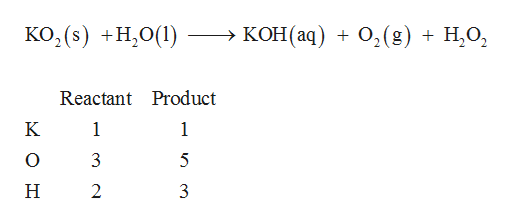# How to balance this chemical equation?KO2(s) +H2O(l) ----> KOH(aq) + O2(g) + H2O2

Question

How to balance this chemical equation?

KO2(s) +H2O(l) ----> KOH(aq) + O2(g) + H2O2

check_circle

Step 1

Balanced chemical equation of a reaction is written according to law of conservation of mass. Thus, in a balanced equation the number of atoms of each element as a reactant is equal to the number of atoms of that eleme...help_outlineImage TranscriptioncloseKO2(s) H,O( - > КОН (аq) + о, (g) + Н,О, Reactant Product K 1 1 3 5 2 3 fullscreen

### Want to see the full answer?

See Solution

#### Want to see this answer and more?

Solutions are written by subject experts who are available 24/7. Questions are typically answered within 1 hour.*

See Solution
*Response times may vary by subject and question.
Tagged in

### General Chemistry